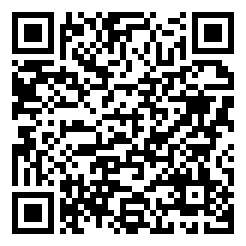Jimmy Zhang Aug 19, 2017
•"与"运算 AND：逻辑乘法

$A$$B$$A \land B$
000
010
100
111

"或"运算 OR：逻辑加法

$A$$B$$A \lor B$
000
011
101
111

"非"运算 NOT：逻辑否定

$A$$\lnot A$
01
10

"与非"运算 NAND

X NAND Y = NOT (X AND Y) == (NOT X) AND (NOT Y)

$A$$B$$\lnot (A \land B)$
001
011
101
110

"或非"运算 NOR

X NOR Y = NOT (X OR Y) == (NOT X) OR (NOT Y)

$A$$B$$\lnot (A \lor B)$
001
010
100
110

"异或"运算 XOR

X XOR Y = ((NOT X) AND Y) OR (X AND (NOT Y))

$A$$B$$A \oplus B$
000
011
101
110

"同或"运算 XNOR

X XNOR Y = ((NOT X) AND (NOT Y) OR (X AND Y)

$A$$B$$A \odot B$
001
010
100
111

• 二进制 Binary
• 八进制 Octal
• 十进制 Decimal

1. 二进制运算规则简单；
2. 二进制算术运算可与逻辑运算实现统一，即可以用逻辑运算实现算术运算；
3. 能表示两种状态的元器件容易找到，如继电器开关、灯泡、二极管/三极管等。

$-1$ 的原码为 $1000 \ 0001$，反码为 $1111 \ 1110$ ，补码为 $1111 \ 1111$ ，移码为 $0111 \ 1111$

ABSUMCARRY
0000
0110
1010
1101

$\begin{cases}$
S_i = A_i \oplus B_i \
C_{i + 1} = A_i \land B_i
\end{cases}

$\begin{cases}$
S_i = (A_i \oplus B_i) \oplus C_i \
C_{i + 1} = ((A_i \oplus B_i) \land C_i) \lor (A_i \land B_i)
\end{cases}

$\sum\limits_{i=0}^{k}a \cdot 2^n$

1. 从被除数的最高位 5 开始，从 0 ~ 9 选一个数，使得 $5 - i \times 3 \ge 0$ 且使 $5 - (i + 1) \times 3 < 0$。我们选择了1，余数为2。
2. 将余数$2 \times 10 + 1 = 21$，继续从 0 ~ 9 中选一个数，使得$21 - 3 \times i \ge 0$且使$5 - (i + 1) \times 3 < 0$。我们选择了7。
3. 由此，我们找到了答案17。

1. 从第一位开始为1，小于11，结果位置0；余数为1
2. 从第二位开始，余数 $1 \times 2 + 1=11$，等于11，结果位置 1，余数为 0；
3. 从第三、四位开始，余数 $0 \times 2 + 0 = 0 < 011$，结果位置 0，余数为 0；
4. 从第5位开始，余数 $0 \times 2 + 1 = 1 < 11$，结果位置 0，余数为 1；
5. 从第6位开始，余数 $1 \times 2 + 1 = 11 = 11$，结果位置 1，余数为 0。

• 唯一性：每种组合都有唯一确定含义。
• 公共性：所有相关者均认同、遵守、使用该种编码。
• 规律性：具有一定规律和一定编码规则，便于计算机和人使用它。

ASCII 码、Unicode 和 GB2312-1980 不在本文中介绍，详情咨询百科。

$1000$ 篇大新闻，其中一篇是长者的，香港记者只要报道了长者的新闻生命就会在24h内被续掉，问至少要多少名香港记者才能在 $24h$ 内鉴别出长者的新闻？

【猪】：这还不简单，$1000$ 名就够啦。

【人】：猪就是蠢，$999$ 名不就行啦。

【犇】：其实 $10$ 名就够了。这其实是一个二进制转十进制问题。我们以 $0$ 代表记者存活，$1$ 代表记者被续。给记者编号 $0 \sim 9$；给新闻编号 $0 \sim 999$

1. $9$ 号记者报道新闻 $512 \sim 999$$2^9$）；
2. $8$ 号记者报道新闻 $256 \sim 51, 768 \sim 999$$2^8$）；
3. $7$ 号记者报道新闻 $128 \sim 255, 384 \sim 511, 640 \sim 767, 896 \sim 999$$2^7$）；
4. $6$ 号记者报道新闻 $64 \sim 127, 192 \sim 255, 320 \sim 383, \dots, 936 \sim 999$$2^6$）；
……
依此类推，直到最后让 $0$ 号记者报道所有的奇数新闻（$2^0$）。

$24h$ 后，将 $0 \sim 9$ 号记者的生存情况排列成一个二进制数，将其转换为 $10$ 进制，即为长者新闻编号。

$1100110001_2 = 817_{10}$

$1000$ 篇新闻，有一篇是能送命的，无非意味着一共仅有 $1000$ 种状态。我们只要找出一种方法能够表示这种状态就可以了——比如 $10$ 位二进制就是一个不错的选择。

This blog is under a CC BY-NC-SA 4.0 Unported License.